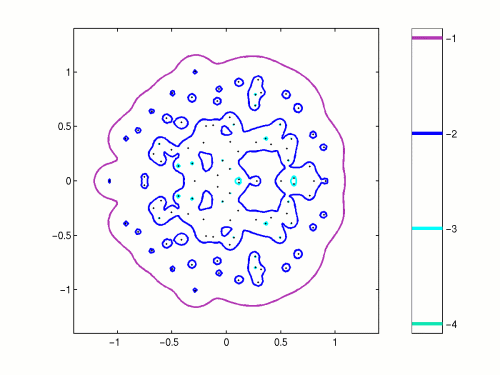Home | Introduction | Software | Examples | History | People | Bibliography | Applications | Theorems

## Pseudospectra of a Dense Random Matrix Dimension N=200Pseudospectra of a random matrix whose entries are drawn from the normal distribution with mean 0 and variance 1/N. This example was first illustrated in [Tre92].

Further details about this example can be found on the page:
Pseudospectra of Random Matrices: Dense and Triangular Matrices.

Use the following MATLAB code compute a similar image using EigTool.

```   N = 200;
A = randn(N)/sqrt(N);
opts.npts = 20;
opts.ax = [-1.5 1.5 -1.5 1.5];
opts.levels = [-4:-1];
eigtool(A, opts)
```
Download this code: randense.m.